# Chapter 3 Pair of Linear Equations in Two Variables Important Questions for CBSE Class 10 Maths Board Exams

This page contains Important Questions for Class 10 Maths Chapter 3 Pair of Linear Equations in Two Variables, which will help the students to prepare for the CBSE Class 10 maths Board exam 2022-23. It help the doing better in their maths paper. Extra questions of Chapter 3 Pair of Linear Equations in Two Variables given here which are based on the pattern of CBSE NCERT book. Students will learn about the entire syllabus and learn how to solve problems in preparation for the exams.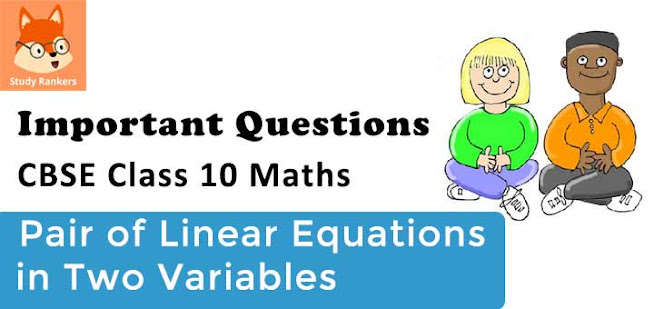## Important Questions for Chapter 3 Pair of Linear Equations in Two Variables Class 10 Maths

Important Questions Class 10 Maths contains all types of questions which could be asked in the examination. We have included short answer questions as well as long answer questions for Chapter 3 Pair of Linear Equations in Two Variables. For more exercise, students can also refer to the Pair of Linear Equations in Two Variables NCERT Solutions. Students can solve these questions and check their answers on the website. All the questions are solved in detail.

### Pair of Linear Equations in Two Variables Class 10 Maths Important Questions Very Short Answer (1 Mark)

1. Find whether the pair of linear equations y = 0 and y = -5 has no solution, unique solution or infinitely many solutions.
Solution
The given variable y has different values. Therefore the pair of equations y = 0 and y = -5 has no solution.

2. If , am = bl then find whether the pair of linear equations ax + by = c and lx + my = n has no
solution, unique solution or infinitely many solutions.
Solution
Since, am = bl, we have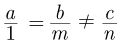Thus, ax by c + = and lx my n + = has no solution.

3. If , ad ≠bc then find whether the pair of linear equations ax + by = p and cx + dy = q has no solution,
unique solution or infinitely many solutions.
Solution
Since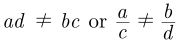Hence, the pair of given linear equations has unique solution.

4. How many solutions does the pair of equations y = 0 and y = -5 have?
Solution
y = 0 and y = -5 are Parallel lines, hence no solution.

5. Two lines are given to be parallel. The equation of one of the lines is 4x + 3y = 14, then find the equation of the second line.

Solution

The equation of one line is 4x + 3y = 14.

We know that if two lines a1x + b1y + c = 0 and a2x + b2y + c = 0 are parallel, then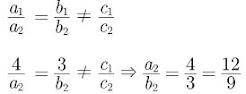Hence, one of the possible, second parallel line is 12x + 9y = 5.

6. If ax + by = a2 – b2 and bx + ay = 0, find the value of (x + y).
Solution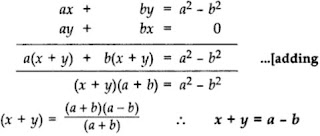7. Calculate the area bounded by the line x + y = 10 and both the co-ordinate axes. (2012)

Solution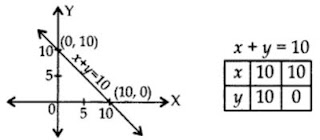Area of triangle
= 12 × base × corresponding altitude
= 12 × 10 × 10 = 50 cm2

8. If the equations kx - 2y = 3 and 3x + y = 5= represent two intersecting lines at unique point, then the value of k is ........... .

Solution

For unique solution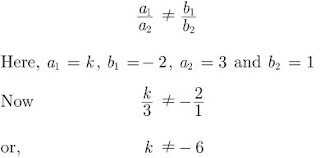9. If the lines are parallel, then the pair of equations has no solution. In this case, the pair of equations is .....

Solution

inconsistent

10. If a pair of linear equations has solution, either a unique or infinitely many, then it is said to be ......

Solution

consistent

### Pair of Linear Equations in Two Variables Class 10 Maths Important Questions Short Answer-I (2 Marks)

11. Solve by elimination:

3x – y – 7
2x + 5y + 1 = 0

Solution

3x – y = 7 …(i)
2x + 5y = -1 -00
Multiplying equation (i) by 5 & (ii) by 1,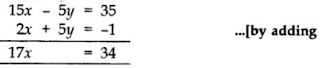⇒ x = 2
Putting the value of x in (i), we have
3(2)-y = 7
⇒ 6 – 7 = y

∴ y = -1 ∴ x = 2, y = -1

12. Find the value(s) of k so that the pair of equations x + 2y = 5 and 3x + ky + 15 = 0 has a unique solution.

Solution

We have,

x + 2y - 5 = 0 ...(1)

and 3x + ky + 15 = 0 ...(2)

Comparing equation (1) with a1x + b1y + c1 = 0 and equation (2) with a2x + b2y + c2 = 0, we get

a1 = 1, a2 = 2, b1 = 2, b2 = k, c1 = -5, c2 = 15

Since, given equations have unique solution, So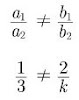k ≠ 6

Hence, for all values of k except 6, the given pair of equations have unique solution.

13. If 2x + y = 23 and 4x - y = 19, find the value of (5y - 2x) and (y/x - 2).

Solution

We have,

2x + y = 23 ...(1)

4x - y = 19 ...(2)

Adding equation (1) and (2), we have

6x = 42

⇒ x = 7

Substituting the value of x in equation (1), we get

14 + y =23

⇒ y = 23 - 14 = 9

Hence,

5y - 2x = 5×9 - 2×7

= 45 - 14

= 31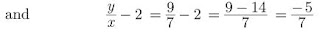14. Find whether the following pair of linear equations is consistent or inconsistent:
3x + 2y = 8 6x – 4y = 9

Solution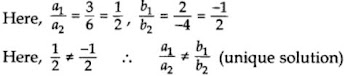Therefore, given pair of linear equations is consistent.

15. Draw the graph of

2y = 4x – 6; 2x = y + 3 and determine whether this system of linear equations has a unique solution or not.

Solution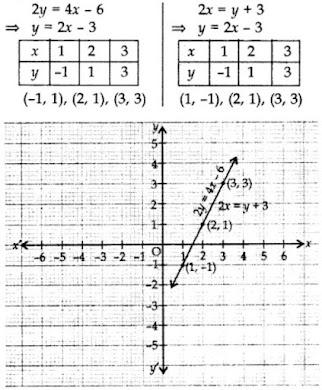Since both the lines coincide.
Therefore infinitely many solutions.

16. Find whether the lines represented by 2x + y = 3 and 4x + 2y = 6 are parallel, coincident or intersecting.

Solution

Here,

a1 = 2, b1 = 1, c1 = -3 and a2 = 4, b2 = 2, c2 = -6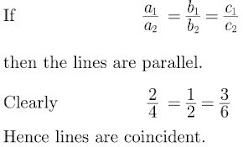17.  Find whether the following pair of linear equation is consistent or inconsistent:

3x + 2y= 8, 6x - 4y= 9

Solution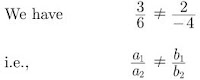Hence, the pair of linear equation is consistent.

18. Is the system of linear equations 2x+ 3y - 9 = 0 and 4x+ 6y - 18 = 0 consistent? Justify your answer.

Solution

For the equation, 2x+ 3y - 9 = 0 we have

a2 = 2, b1 = 3 and c1 = -9 and

for the equation, 4x+ 6y - 18 = 0 we have

a2 = 4, b2 = 6 and c2 = -18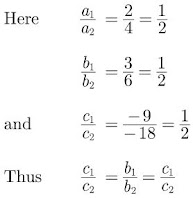Hence, system is consistent and dependent.

### Pair of Linear Equations in Two Variables Class 10 Maths Important Questions Short Answer-II (3 Marks)

19. Solve the following system of equations.
21/x + 47/y = 110, 47/x + 21/y = 162, x, y ≠ 0
Solution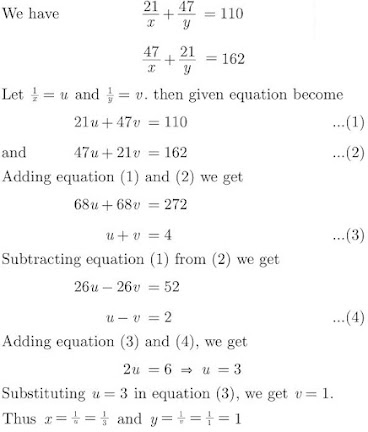20. A fraction becomes 1/3 when 2 is subtracted from the numerator and it becomes 1/2 when 1 is subtracted from the denominator. Find the fraction.
Solution
Let the fraction be x/y. According to the first condition,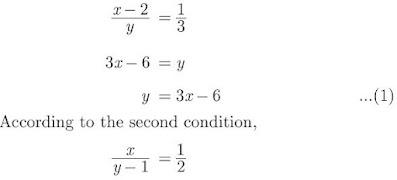2x = y - 1
⇒ y  = 2x + 1 ...(2)
From equation (1) and (2), we have
3x - 6 = 2x + 1
⇒ x = 7
Substitute value of x in equation (1), we get
y = 3(7) - 6
= 21 - 6
= 15
Hence, fraction is 7/15

21. Solve the following pair of equations for x and y:
a2/x − b2/y = 0; a2b/x + b2a/y = a + b, x ≠ 0; y ≠ 0

Solution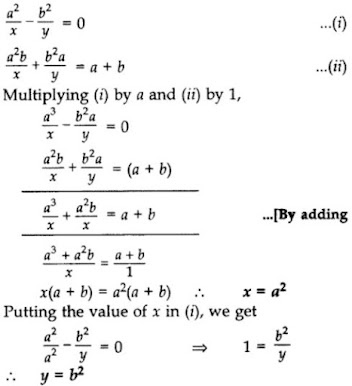22. In the figure, ABCDE is a pentagon with BE||CD and BC||DE. BC is perpendicular to CD. AB = 5cm, AE = 5 cm, BE = 7 cm, BC = x - y and CD = x + y. If the perimeter of ABCDE is 27 cm. Find the value of x and y, given x, y ≠ 0.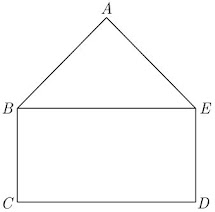Solution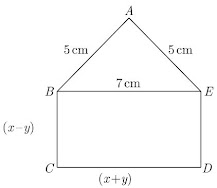We have redrawn the given figure as shown below.
We have,
CD = BE
x + y = 7 ...(1)
Also, perimeter of ABCDE is 27 cm, thus
AB + BC + CD + DE + AE = 27
5 + (x-y) + (x+y) + (x-y) + 5 =27
3x - y = 17 ...(2)
Adding equation (1) and (2) we have
4x = 24
x = 6
Substituting x = 6 in equation (1) we obtain
y = 7 - x = 7-6 = 1
Thus x = 6 and y = 1

23. Given the linear equation 2x + 3y - 8 = 0, write another linear equation in two variables such that the geometrical representation of the pair so formed is :
(a) intersecting lines
(b) parallel lines
(c) coincident lines.
Solution
Given, linear equation is 2x + 3y - 8 = 0 ...(1)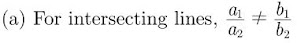To get its parallel line one of the possible equation may be taken as
5x + 2y - 9 = 0 ...(2)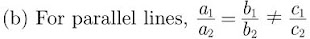One of the possible line parallel to equation (1) may be taken as
6x + 9y + 7 = 0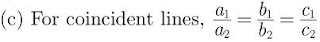To get its coincident line, one of the possible equation may be taken as
4x + 6y -16 = 0

24. Determine the values of m and n so that the following system of linear equation have infinite number of solutions :

(2m - 1)x + 3y - 5 = 0

3x + (n-1)y - 2 = 0

Solution

We have (2m -1)x + 3y - 5 = 0 ...(1)

Here, a1 = 2m -1, b1 = 3, c1 = -5

3x + (n-1)y - 2 = 0 ...(2)

Here, a2 = 3, b2 = (n-1), c2 = -2

For a pair of linear equations to have infinite number of solutions,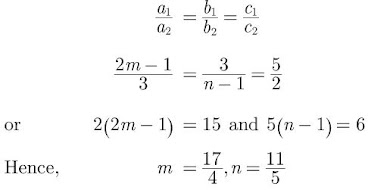25. Find the values of α and β for which the following pair of linear equations has infinite number of solutions :
2x + 3y = 7, 2αx + (α+β)y = 28
Solution
We have 2x + 3y = 7 and 2αx + (α+β)y = 28. For a pair of linear equations to be consistent and having infinite number of solutions,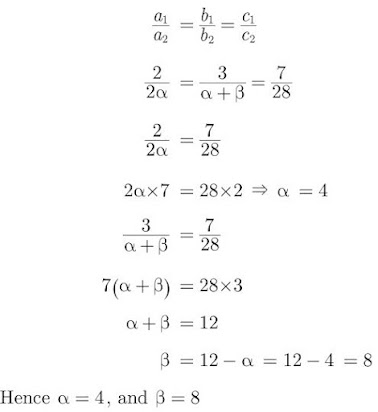26. Solve for x and y:
10/x+y + 2/x−y = 4; 15/x+y − 5/x−y = −2
x + y ≠ 0
x – y ≠ 0
Solution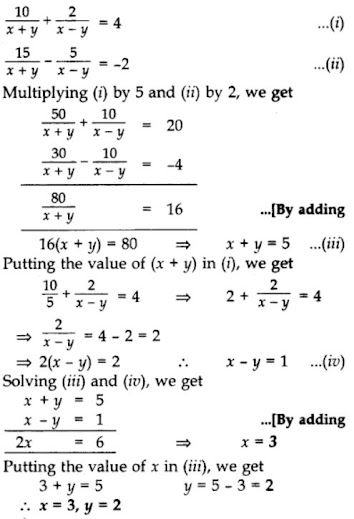27. Solve by elimination:
3x = y + 5
5x – y = 11
Solution
We have, 3x = y + 5, and 5x – y = 11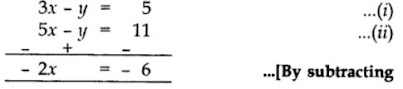Putting the value of x in (i), we get
3x – y = 5
⇒ 3(3) – y = 5
9 – 5 = y
⇒ y = 4
∴ x = 3, y = 4

28. Solve for x and y:
27x + 31y = 85;
31x + 27y = 89
Solution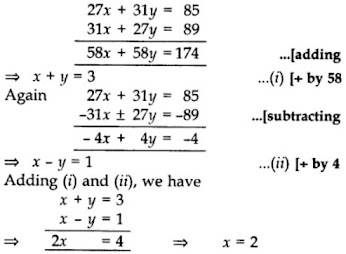Putting the value of ‘x’ in (i), we get
2 + y = 3
⇒ y = 3 – 2 = 1
∴ x = 2, y = 1

29. Solve for x and y: x/a - y/b = 0;

ax + by = a2+ b2

Solution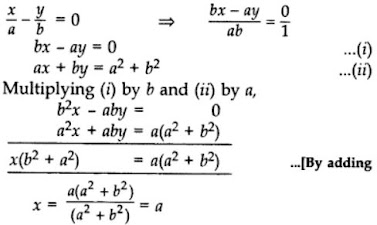Putting the value of x in (i), we get

b(a) – ay = 0

⇒ ba = ay

ba/a = y

∴ b = y

∴ x = a, y = b

30. Find the two numbers whose sum is 75 and difference is 15.

Solution

Let the two numbers be x and y.

According to the question,

x + y = 75 …(i)

∴ x – y = ±15 …(ii)

Solving (i) and (ii), we get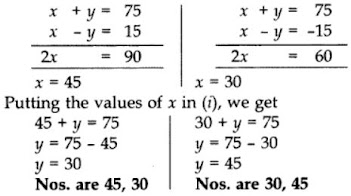31. Sum of the ages of a father and the son is 40 years. If father’s age is three times that of his son, then find their respective ages.
Solution
Let age of father and son be x and y respectively.
x + y = 40 ...(1)
x = 3y ...(2)
Solving equations (1) and (2), we get
x = 30 and y = 10
Ages are 30 years and 10 years.

32. The sum of the digits of a two digit number is 8 and the difference between the number and that formed by reversing the digits is 18. Find the number. (2015)

Solution

Let unit and tens digit be x and y.

∴ Original number = 1x + 10y …(i)
Reversed number = 10x + 1y
According to question,
x + y = 8
⇒ y = 8 – x …(ii)
Also, 1x + 10Oy – (10x + y) = 18
⇒ x + 10y – 10x – y = 18
⇒ 9y – 9x = 18
⇒ y – x = 2 …[Dividing both sides by 9]
⇒ 8 – x – x = 2 …[From (ii)]
⇒ 8 – 2 = 2x
⇒ 2x = 6
From (it), y = 8 – 3 = 5
From (i), Original number = 3 + 10(5) = 53

### Pair of Linear Equations in Two Variables Class 10 Maths Important Questions Long Answer (4 Marks)

33. A man can row a boat downstream 20 km in 2 hours and upstream 4 km in 2 hours. Find his speed of rowing in still water. Also find the speed of the stream.
Solution
Let x be the speed of the boat in still water and y be the speed of the stream.
Relative Speed of boat in upstream will be (x - y) and relative speed of boat in downstream will be (x + y).
According to question, we have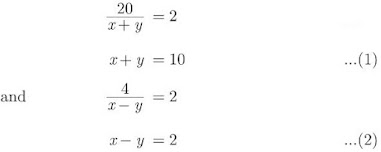Adding equation (1) and (2), we have
2x = 12
⇒ x = 6 km/hr
Substituting the value of x is equation (1) we have,
6 + y = 10
⇒ y = 10 - 6 = 4 km/hr
Thus speed of a boat in still water is 6 km/hr and
speed of the stream 4 km/hr.

34. It can take 12 hours to fill a swimming pool using two pipes. If the pipe of larger diameter is used for four hours and the pipe of smaller diameter for 9 hours, only half of the pool can be filled. How long would it take for each pipe to fill the pool separately?
Solution
Let x be time taken to fill the pool by the larger diameter pipe and y be the time taken to fill the pool by the smaller diameter pipe.
According to question,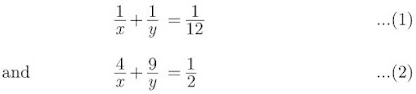Multiplying equation (1) by 9 and subtracting from equation (2), we get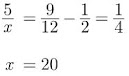Substituting the value of x in equation (1), we have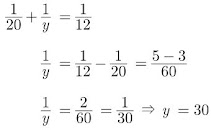Hence, time taken to fill the pool by the larger and smaller diameter pipe are 20 hrs and 30 hrs respectively.

35. Amit bought two pencils and three chocolates for ₹11 and Sumeet bought one pencil and two chocolates for ₹7. Represent this situation in the form of a pair of linear equations. Find the price of one pencil and that of one chocolate graphically.

Solution

Let the price of one pencil = ₹x and the price of one chocolate = ₹y.
As per the Question,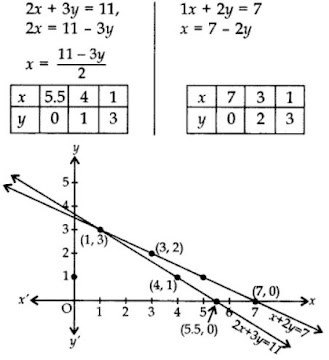Lines intersect at (1, 3).

∴ x = 1, y = 3

Therefore the price of one pencil = ₹1 and price of one chocolate = ₹3

36. Draw the graphs of following equations:

2x – y = 1; x + 2y = 13

Find the solution of the equations from the graph and shade the triangular region formed by the lines and the y-axis. (2013)

Solution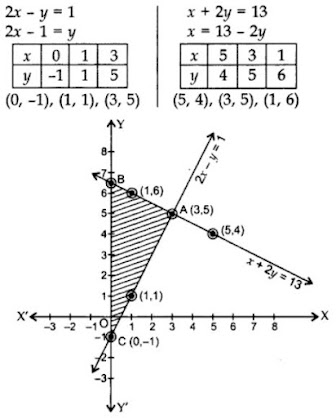By plotting the points and joining them, the lines intersect at A(3,5).

∴ x = 3, y = 5

Here ∆ABC is the required triangle.

37. For what value of k, which the following pair of linear equations have infinitely many solutions:

2x + 3y = 7 and (k+1)x + (2k-1)y = 4k + 1

Solution

We have,

2x + 3y = 7

and (k+1)x + (2k-1)y = 4k + 1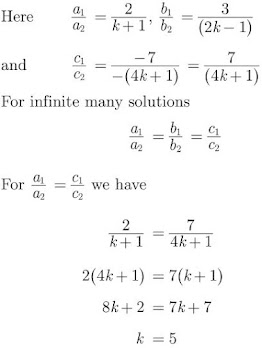Hence, the value of k is 5, for which the given equation have infinitely many solutions.

38. The owner of a taxi company decides to run all the taxis on CNG fuel instead of petrol/diesel. The taxi charges in city comprises of fixed charges together with the charge for the distance covered. For a journey of 13 km, the charge paid is ₹129 and for a journey of 22 km, the charge paid is ₹210. What will a person have to pay for travelling a distance of 32 km?

Solution

Let fixed charge be ₹x and the charge for the distance = ₹y per km
According to the Question,
For a journey of 13 km,
x + 13y = 129
⇒ x = 129 – 13y …(i)

For a journey of 22 km,
x + 22y = 210 …(ii)
⇒ 129 – 13y + 22y = 210 …[From (i)]
⇒ 9y = 210 – 129 = 81
⇒ 9y = 81
⇒ y = 9

From (i),
x = 129 – 13(9)
= 129 – 117 = 12
∴ Fixed charge, x = ₹12
∴ The charge for the distance, y = ₹9 per km
To pay for travelling a distance of 32 km
= x + 32y = 12 + 32(9) = 12 + 288 = ₹300